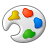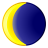# How are p-value for Anselin LISA and Getis-Ord's G statistics computed?

3903
2
Jump to solution
05-18-2014 05:07 PMby
New Contributor
is monte carlo simulation used in place of normal approximation? I can't find any mention of monte carlo on the tools' help pages. In fact what i found appears to be conflicting documentations. According to your documentation for Getis-Ord's G for example, '...The Z scores are reliable (even with skewed data) as long as each feature is associated with several neighbors (approximately 8, as a rule of thumb). This tool can be applied to skewed data because it is "asymptotically normal". ' This suggests p-values were computed usig normal approximation. But on your p-value documentation page, you say 'A common alternative null hypothesis, not implemented for the spatial statistics toolbox, is the normalization null hypothesis. The normalization null hypothesis postulates that the observed values are derived from an infinitely large, normally distributed population of values through some random sampling process.'  I am really confused as to what method is used to get the p-values for LISA and local G statistics and would apreciate any clarification! thx!
Tags (2)
1 Solution

Accepted SolutionsOccasional Contributor
At present the z-scores are computed using the mathematics that we�??ve documented (http://resources.arcgis.com/en/help/main/10.2/index.html#/How_Hot_Spot_Analysis_Getis_Ord_Gi_works/0..., http://resources.arcgis.com/en/help/main/10.2/index.html#/How_Cluster_and_Outlier_Analysis_Anselin_L...).  These formulas were obtained from the seminal articles about the methods (the articles are listed below).  We are not using Monte Carlo methods and, at present, are not computing z-scores using permutation (conditional randomization).  Our z-score calculations are based on the randomization null hypothesis (theoretical distribution)�?�  they are not based on simulation or permutation.

P-values have a one to one correspondence with z-scores (i.e., a z-score of + or - 1.96 will always equate to a p-value of 0.05).  Our tools calculate z-scores and then translate those z-scores to p-values.  Our tools report both z-score and p-value results.

Our empirical tests support the seminal work on Gi* by Getis and Ord who, in their 1992 paper, show that the statistic is asymptotically normal.  Z-Scores do have a normal distribution so often people will ask us if it is valid to run Hot Spot Analysis (Gi*) on data that is skewed.  The answer is yes, as long as the threshold distance you use is not too small or too large.  How do we know?  We start with very skewed data sets (like crime counts) and then compare the calculated p-values, based on the asymptotic z-scores, to the pseudo p-values obtained from permutations (conditional randomization).  We found that for as low as 16 neighbors the asymptotic results provided the same significance as the permutations did over 99.9% of the time.  We tested this on over 10 different skewed data sets, including mixed discrete/continuous models.

In Anselin�??s article (citation below, page 99), the mathematics for calculated z-scores based on the randomization null hypothesis is given (equations 13, 14, and appendix A).  The author indicates that a test for significant local spatial association may be based on these equations, but notes that the exact distribution is unknown.  He suggests a conditional randomization alternative.  Our empirical testing confirms that the permutation approach will be more accurate for this statistic when data is skewed; the Local Moran�??s I statistic does not appear to be asymptotically normal.  We have already begun the development work to compute z-scores using permutation and will put this functionality in to the next release of ArcGIS.

With the 10.1.2 release of ArcGIS we added a False Discovery Rate (FDR) p-value correction.  We still report the uncorrected z-scores and p-values but use the correction to account for multiple testing and spatial dependency.  For more about the FDR correction, please see:
http://resources.arcgis.com/en/help/main/10.2/#/What_is_a_z_score_What_is_a_p_value/005p000000060000...

Here are some additional resources:
�?� 1992 Getis and Ord paper: http://onlinelibrary.wiley.com/doi/10.1111/j.1538-4632.1992.tb00261.x/abstract
�?� 1995 Ord and Getis paper (this is the version of the Gi* we implement):  http://onlinelibrary.wiley.com/doi/10.1111/j.1538-4632.1995.tb00912.x/abstract
�?� Seminal Anselin paper used as a basis for our Cluster and Outlier Analysis tool:  Anselin, Luc.  �??Local Indicators of Spatial Association �?? LISA.�?�  Geographical Analysis Vol 27, no 2 (April 1995): 93-115.
�?� Very good article about FDR: Caldas de Castro, Marcia, and Burton H. Singer. "Controlling the False Discovery Rate: A New Application to Account for Multiple and Dependent Test in Local Statistics of Spatial Association." Geographical Analysis 38, pp 180-208, 2006.

Please let me know if I have not answered your question.
Best wishes,
Lauren

Lauren M Scott, PhD
Esri
Geoprocessing, Spatial Statistics
2 RepliesOccasional Contributor
At present the z-scores are computed using the mathematics that we�??ve documented (http://resources.arcgis.com/en/help/main/10.2/index.html#/How_Hot_Spot_Analysis_Getis_Ord_Gi_works/0..., http://resources.arcgis.com/en/help/main/10.2/index.html#/How_Cluster_and_Outlier_Analysis_Anselin_L...).  These formulas were obtained from the seminal articles about the methods (the articles are listed below).  We are not using Monte Carlo methods and, at present, are not computing z-scores using permutation (conditional randomization).  Our z-score calculations are based on the randomization null hypothesis (theoretical distribution)�?�  they are not based on simulation or permutation.

P-values have a one to one correspondence with z-scores (i.e., a z-score of + or - 1.96 will always equate to a p-value of 0.05).  Our tools calculate z-scores and then translate those z-scores to p-values.  Our tools report both z-score and p-value results.

Our empirical tests support the seminal work on Gi* by Getis and Ord who, in their 1992 paper, show that the statistic is asymptotically normal.  Z-Scores do have a normal distribution so often people will ask us if it is valid to run Hot Spot Analysis (Gi*) on data that is skewed.  The answer is yes, as long as the threshold distance you use is not too small or too large.  How do we know?  We start with very skewed data sets (like crime counts) and then compare the calculated p-values, based on the asymptotic z-scores, to the pseudo p-values obtained from permutations (conditional randomization).  We found that for as low as 16 neighbors the asymptotic results provided the same significance as the permutations did over 99.9% of the time.  We tested this on over 10 different skewed data sets, including mixed discrete/continuous models.

In Anselin�??s article (citation below, page 99), the mathematics for calculated z-scores based on the randomization null hypothesis is given (equations 13, 14, and appendix A).  The author indicates that a test for significant local spatial association may be based on these equations, but notes that the exact distribution is unknown.  He suggests a conditional randomization alternative.  Our empirical testing confirms that the permutation approach will be more accurate for this statistic when data is skewed; the Local Moran�??s I statistic does not appear to be asymptotically normal.  We have already begun the development work to compute z-scores using permutation and will put this functionality in to the next release of ArcGIS.

With the 10.1.2 release of ArcGIS we added a False Discovery Rate (FDR) p-value correction.  We still report the uncorrected z-scores and p-values but use the correction to account for multiple testing and spatial dependency.  For more about the FDR correction, please see:
http://resources.arcgis.com/en/help/main/10.2/#/What_is_a_z_score_What_is_a_p_value/005p000000060000...

Here are some additional resources:
�?� 1992 Getis and Ord paper: http://onlinelibrary.wiley.com/doi/10.1111/j.1538-4632.1992.tb00261.x/abstract
�?� 1995 Ord and Getis paper (this is the version of the Gi* we implement):  http://onlinelibrary.wiley.com/doi/10.1111/j.1538-4632.1995.tb00912.x/abstract
�?� Seminal Anselin paper used as a basis for our Cluster and Outlier Analysis tool:  Anselin, Luc.  �??Local Indicators of Spatial Association �?? LISA.�?�  Geographical Analysis Vol 27, no 2 (April 1995): 93-115.
�?� Very good article about FDR: Caldas de Castro, Marcia, and Burton H. Singer. "Controlling the False Discovery Rate: A New Application to Account for Multiple and Dependent Test in Local Statistics of Spatial Association." Geographical Analysis 38, pp 180-208, 2006.

Please let me know if I have not answered your question.
Best wishes,
Lauren

Lauren M Scott, PhD
Esri
Geoprocessing, Spatial Statisticsby
New Contributor
Thank you so much Lauren for the detailed explanations. This is exactly the kind of information I am looking for! Thanks!!